# Customizable correlation plots in R

programming
Author

Katherine Hoffman

Published

August 24, 2020

Updated to include `gt` package August 23, 2022.

If you’re ever felt limited by correlogram packages in `R`, this post will show you how to write your own function to tidy the many correlations into a `ggplot2`-friendly form for plotting.

By the end of this post, you will be able to run one function to get a tidied data frame of correlations:

``formatted_cors(mtcars) %>% head() %>% gt()``
measure1 measure2 r n p sig_p p_if_sig r_if_sig
mpg mpg 1.0000000 32 NA NA NA NA
mpg cyl -0.8521620 32 6.112688e-10 TRUE 6.112688e-10 -0.8521620
mpg disp -0.8475514 32 9.380328e-10 TRUE 9.380328e-10 -0.8475514
mpg hp -0.7761684 32 1.787835e-07 TRUE 1.787835e-07 -0.7761684
mpg drat 0.6811719 32 1.776240e-05 TRUE 1.776240e-05 0.6811719
mpg wt -0.8676594 32 1.293958e-10 TRUE 1.293958e-10 -0.8676594

You can then run `ggplot2` code on this data to make your own correlation heat maps.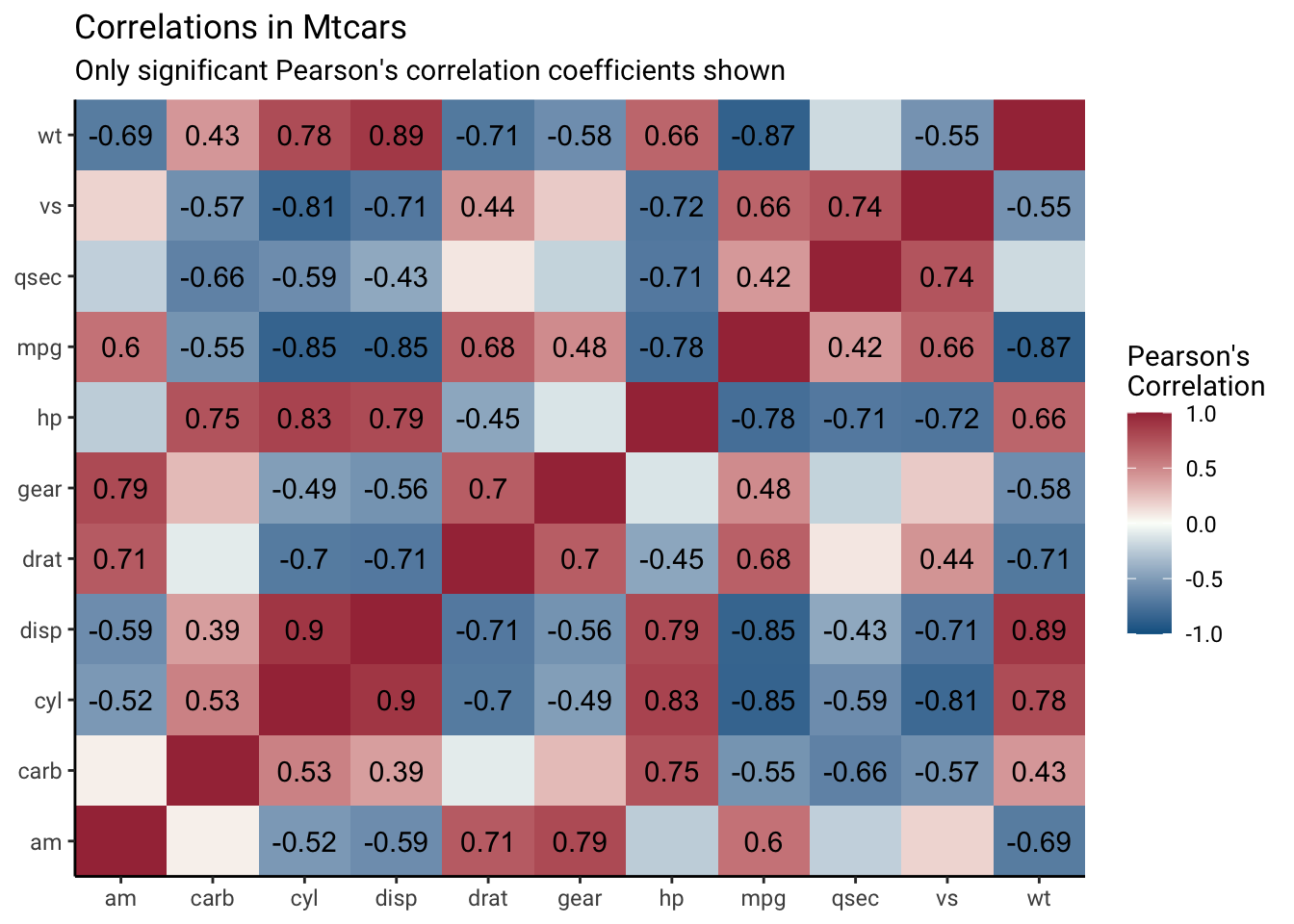# Less-customizable options

I really appreciate some of the packages and functions that allow me to make correlation plots super quickly using `R`. Here are a few examples:

``corrplot::corrplot(cor(mtcars))``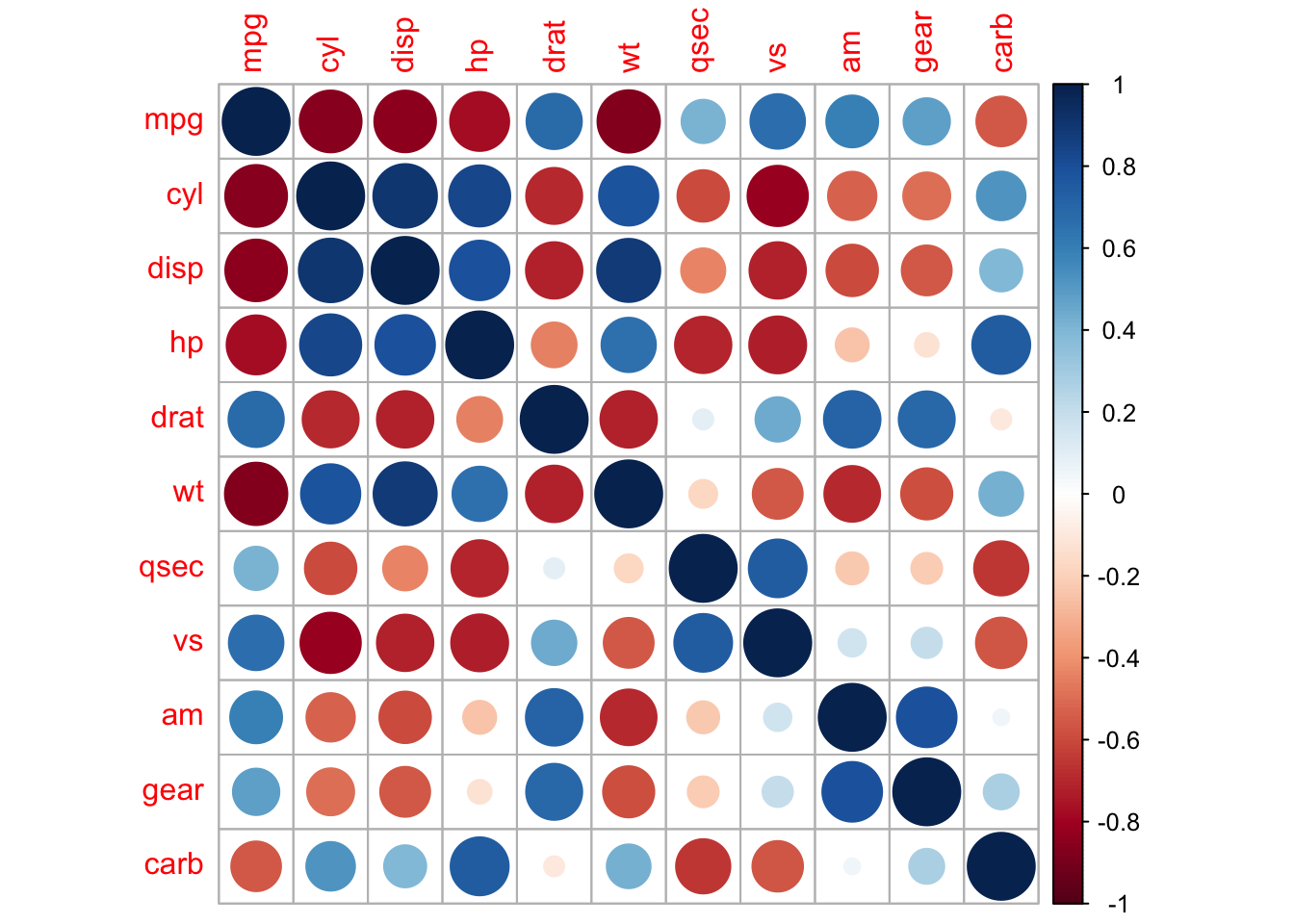``corrgram::corrgram(mtcars)``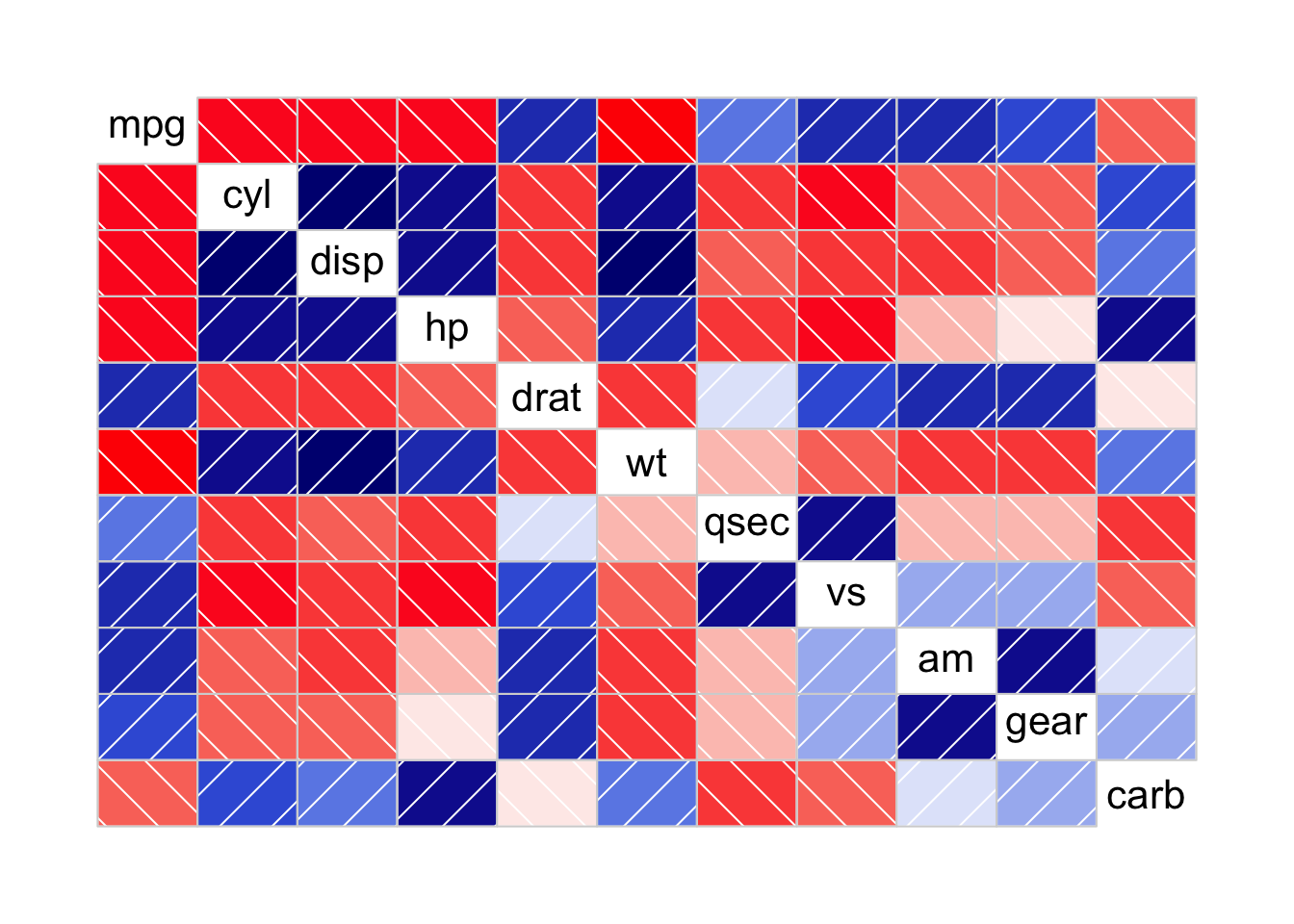``ggcorrplot::ggcorrplot(cor(mtcars))``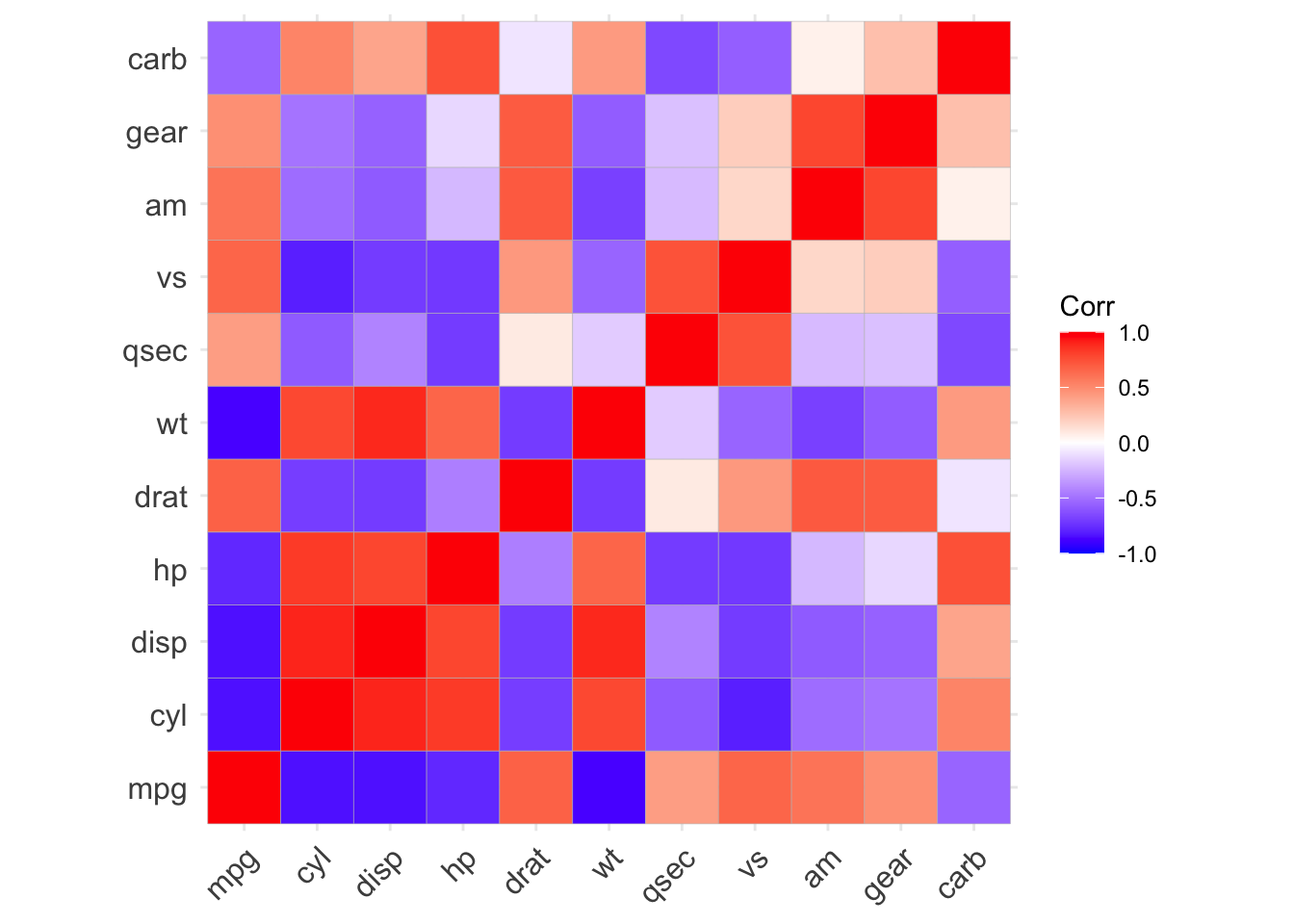All of these are nice, but none of them are ultimately as customizable as I need them to be. I’ll next show how you can bypass using someone else’s function constraints to prepare correlations in your data in a `ggplot2`-friendly format.

# Getting the correlations

We could use the base `R` function `cor()` to get our correlations, but I do not like the defaults for missing data. Instead, I use Frank Harrell’s `Hmisc::rcorr()` function for two reasons:

1. it drops missing pairs as the default

2. it returns p-values, so you only need one function to get both the correlation coefficient and matching p-value

Let’s load the libraries we’ll need for this, which are `knitr` for showing tables using `gt`, and `tidyverse` (we’ll specifically use `tidyr`, `dplyr`, `ggplot2`, `tibble` and `purrr`).

``````library(knitr)
library(gt)
library(tidyverse, warn.conflict=F)``````

First, let’s look at our output from our correlation function we’ll use, `Hmisc::rcorr()`. It requires the input to be a matrix, and outputs a list of three matrices.

``mtcars_cor <- Hmisc::rcorr(as.matrix(mtcars))``

These three matrices include the correlation coefficient (default is Pearson’s), `r`, the p-value, `P`, and the number of observations used for each correlation, `n`. Let’s turn each matrix into a `data frame` and look at the top six rows with `head` and `gt`.

The correlation coefficients, `r`:

``data.frame(mtcars_cor\$r) %>% head() %>% gt()``
mpg cyl disp hp drat wt qsec vs am gear carb
1.0000000 -0.8521620 -0.8475514 -0.7761684 0.6811719 -0.8676594 0.41868403 0.6640389 0.5998324 0.4802848 -0.5509251
-0.8521620 1.0000000 0.9020329 0.8324475 -0.6999381 0.7824958 -0.59124207 -0.8108118 -0.5226070 -0.4926866 0.5269883
-0.8475514 0.9020329 1.0000000 0.7909486 -0.7102139 0.8879799 -0.43369788 -0.7104159 -0.5912270 -0.5555692 0.3949769
-0.7761684 0.8324475 0.7909486 1.0000000 -0.4487591 0.6587479 -0.70822339 -0.7230967 -0.2432043 -0.1257043 0.7498125
0.6811719 -0.6999381 -0.7102139 -0.4487591 1.0000000 -0.7124406 0.09120476 0.4402785 0.7127111 0.6996101 -0.0907898
-0.8676594 0.7824958 0.8879799 0.6587479 -0.7124406 1.0000000 -0.17471588 -0.5549157 -0.6924953 -0.5832870 0.4276059

The p-values, `P`:

``data.frame(mtcars_cor\$P) %>% head() %>% gt()``
mpg cyl disp hp drat wt qsec vs am gear carb
NA 6.112688e-10 9.380328e-10 1.787835e-07 1.776240e-05 1.293958e-10 1.708199e-02 3.415937e-05 2.850207e-04 5.400948e-03 1.084446e-03
6.112688e-10 NA 1.803002e-12 3.477861e-09 8.244636e-06 1.217567e-07 3.660533e-04 1.843018e-08 2.151207e-03 4.173297e-03 1.942340e-03
9.380328e-10 1.803002e-12 NA 7.142679e-08 5.282022e-06 1.222311e-11 1.314404e-02 5.235012e-06 3.662114e-04 9.635921e-04 2.526789e-02
1.787835e-07 3.477861e-09 7.142679e-08 NA 9.988772e-03 4.145827e-05 5.766253e-06 2.940896e-06 1.798309e-01 4.930119e-01 7.827810e-07
1.776240e-05 8.244636e-06 5.282022e-06 9.988772e-03 NA 4.784260e-06 6.195826e-01 1.167553e-02 4.726790e-06 8.360110e-06 6.211834e-01
1.293958e-10 1.217567e-07 1.222311e-11 4.145827e-05 4.784260e-06 NA 3.388683e-01 9.798492e-04 1.125440e-05 4.586601e-04 1.463861e-02

The number of observations, `n`. There are no missing data in the `mtcars` data set so there are 32 pairs used for all correlations.

``data.frame(mtcars_cor\$n) %>% head(n=3) %>% gt()``
mpg cyl disp hp drat wt qsec vs am gear carb
32 32 32 32 32 32 32 32 32 32 32
32 32 32 32 32 32 32 32 32 32 32
32 32 32 32 32 32 32 32 32 32 32

Next we can write a function that formats a `data frame` correctly for `Hmisc::rcorr()` and then turns each of the three elements of the list (`r`,`n` and `P`)

``````cors <- function(df) {
M <- Hmisc::rcorr(as.matrix(df))
# turn all three matrices (r, n, and P into a data frame)
Mdf <- map(M, ~data.frame(.x))
# return the three data frames in a list
return(Mdf)
}``````

Nothing too crazy happened in this function. Now we just have a list of three data frames. We can look at the the first element of our list using `first()`, which shows us the correlations between all our variables:

``cors(mtcars) %>% first() %>% head() %>% gt()``
mpg cyl disp hp drat wt qsec vs am gear carb
1.0000000 -0.8521620 -0.8475514 -0.7761684 0.6811719 -0.8676594 0.41868403 0.6640389 0.5998324 0.4802848 -0.5509251
-0.8521620 1.0000000 0.9020329 0.8324475 -0.6999381 0.7824958 -0.59124207 -0.8108118 -0.5226070 -0.4926866 0.5269883
-0.8475514 0.9020329 1.0000000 0.7909486 -0.7102139 0.8879799 -0.43369788 -0.7104159 -0.5912270 -0.5555692 0.3949769
-0.7761684 0.8324475 0.7909486 1.0000000 -0.4487591 0.6587479 -0.70822339 -0.7230967 -0.2432043 -0.1257043 0.7498125
0.6811719 -0.6999381 -0.7102139 -0.4487591 1.0000000 -0.7124406 0.09120476 0.4402785 0.7127111 0.6996101 -0.0907898
-0.8676594 0.7824958 0.8879799 0.6587479 -0.7124406 1.0000000 -0.17471588 -0.5549157 -0.6924953 -0.5832870 0.4276059

# Prep the correlations for `ggplot2`

The next step is to get the data ready for plotting with `ggplot2`. We can keep the data in a list for now and use the `map()` function from `purrr`.

First, we need to move the rownames to their own column using `tibble::rownames_to_column()`. The output of that looks like:

``````cors(mtcars) %>%
map(~rownames_to_column(.x, var="measure1")) %>%
# look at the first element of the list (r)
first() %>%
gt()``````
measure1 mpg cyl disp hp drat wt qsec vs am gear carb
mpg 1.0000000 -0.8521620 -0.8475514 -0.7761684 0.6811719 -0.8676594 0.41868403 0.6640389 0.5998324 0.4802848 -0.5509251
cyl -0.8521620 1.0000000 0.9020329 0.8324475 -0.6999381 0.7824958 -0.59124207 -0.8108118 -0.5226070 -0.4926866 0.5269883
disp -0.8475514 0.9020329 1.0000000 0.7909486 -0.7102139 0.8879799 -0.43369788 -0.7104159 -0.5912270 -0.5555692 0.3949769
hp -0.7761684 0.8324475 0.7909486 1.0000000 -0.4487591 0.6587479 -0.70822339 -0.7230967 -0.2432043 -0.1257043 0.7498125
drat 0.6811719 -0.6999381 -0.7102139 -0.4487591 1.0000000 -0.7124406 0.09120476 0.4402785 0.7127111 0.6996101 -0.0907898
wt -0.8676594 0.7824958 0.8879799 0.6587479 -0.7124406 1.0000000 -0.17471588 -0.5549157 -0.6924953 -0.5832870 0.4276059

Next, we can turn move of the columns to a single column called `measure2` using `tidyr::pivot_longer()`

``````cors(mtcars) %>%
map(~rownames_to_column(.x, var="measure1")) %>%
# format each data set (r,P,n) long
map(~pivot_longer(.x, -measure1, "measure2")) %>%
# look at the first element of the list (r)
first() %>%
gt()``````
measure1 measure2 value
mpg mpg 1.0000000
mpg cyl -0.8521620
mpg disp -0.8475514
mpg hp -0.7761684
mpg drat 0.6811719
mpg wt -0.8676594

Now, we’re ready to unlist our data by using `bind_rows()`. This will turn our correlations into a very long data frame with all the rows from `r`, then `n`, then `P`.

``````cors(mtcars) %>%
map(~rownames_to_column(.x, var="measure1")) %>%
# format each data set (r,P,n) long
map(~pivot_longer(.x, -measure1, "measure2")) %>%
# merge our three list elements by binding the rows
bind_rows(.id = "id") %>%
gt()``````
id measure1 measure2 value
r mpg mpg 1.0000000
r mpg cyl -0.8521620
r mpg disp -0.8475514
r mpg hp -0.7761684
r mpg drat 0.6811719
r mpg wt -0.8676594

For `ggplot2`, we’ll need to have `r`, `n`, and `p` as their own column. We can use `pivot_longer()` to do this.

``````cors(mtcars) %>%
map(~rownames_to_column(.x, var="measure1")) %>%
# format each data set (r,P,n) long
map(~pivot_longer(.x, -measure1, "measure2")) %>%
# merge our three list elements by binding the rows
bind_rows(.id = "id") %>%
pivot_wider(names_from = id, values_from = value) %>%
gt()``````
measure1 measure2 r n P
mpg mpg 1.0000000 32 NA
mpg cyl -0.8521620 32 6.112688e-10
mpg disp -0.8475514 32 9.380328e-10
mpg hp -0.7761684 32 1.787835e-07
mpg drat 0.6811719 32 1.776240e-05
mpg wt -0.8676594 32 1.293958e-10

Finally, we can add a few columns that will potentially be useful later for making our correlation plots more informative. Let’s add columns that tell us whether the p-value was less than 0.05, and if so, give us back 1) the p-value and 2) the correlation coefficient, in case we want to label our plot with these values.

``````cors(mtcars) %>%
map(~rownames_to_column(.x, var="measure1")) %>%
# format each data set (r,P,n) long
map(~pivot_longer(.x, -measure1, "measure2")) %>%
# merge our three list elements by binding the rows
bind_rows(.id = "id") %>%
pivot_wider(names_from = id, values_from = value) %>%
# change so everything is lower case
rename(p = P) %>%
mutate(sig_p = ifelse(p < .05, T, F),
p_if_sig = ifelse(sig_p, p, NA),
r_if_sig = ifelse(sig_p, r, NA)) %>%
gt()``````
measure1 measure2 r n p sig_p p_if_sig r_if_sig
mpg mpg 1.0000000 32 NA NA NA NA
mpg cyl -0.8521620 32 6.112688e-10 TRUE 6.112688e-10 -0.8521620
mpg disp -0.8475514 32 9.380328e-10 TRUE 9.380328e-10 -0.8475514
mpg hp -0.7761684 32 1.787835e-07 TRUE 1.787835e-07 -0.7761684
mpg drat 0.6811719 32 1.776240e-05 TRUE 1.776240e-05 0.6811719
mpg wt -0.8676594 32 1.293958e-10 TRUE 1.293958e-10 -0.8676594

This seems like everything I think I’ll ever ever want to plot. Of course you could add more. At this point I turned my formatted correlations into a function:

``````formatted_cors <- function(df){
cors(df) %>%
map(~rownames_to_column(.x, var="measure1")) %>%
map(~pivot_longer(.x, -measure1, "measure2")) %>%
bind_rows(.id = "id") %>%
pivot_wider(names_from = id, values_from = value) %>%
rename(p = P) %>%
mutate(sig_p = ifelse(p < .05, T, F),
p_if_sig = ifelse(sig_p, p, NA),
r_if_sig = ifelse(sig_p, r, NA))
}``````

We can test the function works as expected:

``formatted_cors(mtcars) %>% head() %>% gt()``
measure1 measure2 r n p sig_p p_if_sig r_if_sig
mpg mpg 1.0000000 32 NA NA NA NA
mpg cyl -0.8521620 32 6.112688e-10 TRUE 6.112688e-10 -0.8521620
mpg disp -0.8475514 32 9.380328e-10 TRUE 9.380328e-10 -0.8475514
mpg hp -0.7761684 32 1.787835e-07 TRUE 1.787835e-07 -0.7761684
mpg drat 0.6811719 32 1.776240e-05 TRUE 1.776240e-05 0.6811719
mpg wt -0.8676594 32 1.293958e-10 TRUE 1.293958e-10 -0.8676594

# Plotting

We’re finally ready to plot our correlation heat maps in `ggplot2`.

The simplest form of this plot only requires us to specify `measure1` and `measure2` on the `x` and `y`-axis, respectively. Then we can map the correlation `r` to the `fill` `aes`thetic, and add a tile as the `geom`etry.

``````formatted_cors(mtcars) %>%
ggplot(aes(x = measure1, y = measure2, fill = r)) +
geom_tile()``````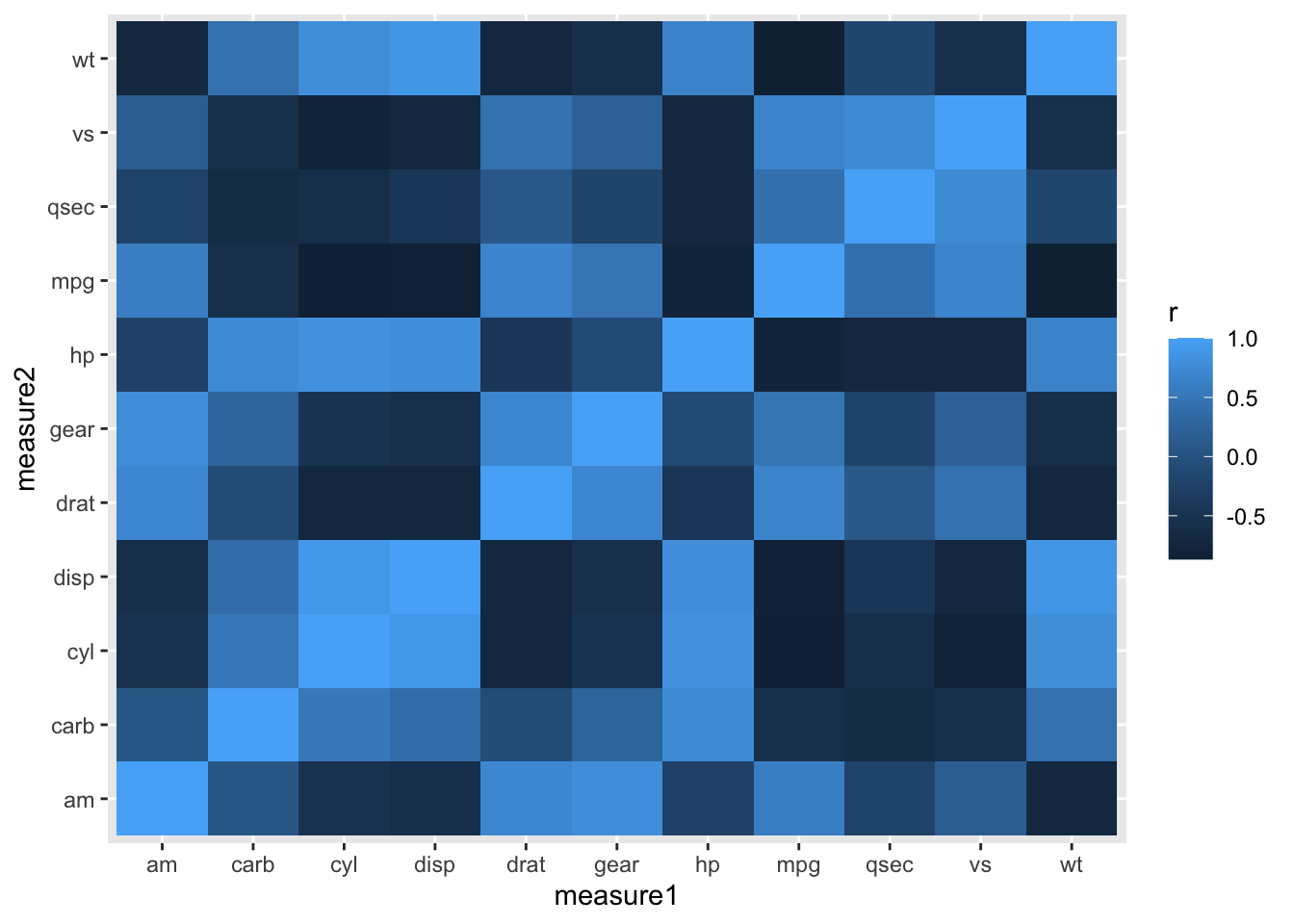We can make some minor aesthetic changes, such as the fill coloring scale, titles, and font family.

``````formatted_cors(mtcars) %>%
ggplot(aes(x = measure1, y = measure2, fill = r)) +
geom_tile() +
labs(x = NULL, y = NULL, fill = "Pearson's\nCorrelation", title="Correlations in Mtcars") +
# map a red, white and blue color scale to correspond to -1:1 sequential gradient
theme_classic() +
# remove excess space on x and y axes
scale_x_discrete(expand=c(0,0)) +
scale_y_discrete(expand=c(0,0)) +
# change global font to roboto
theme(text=element_text(family="Roboto"))``````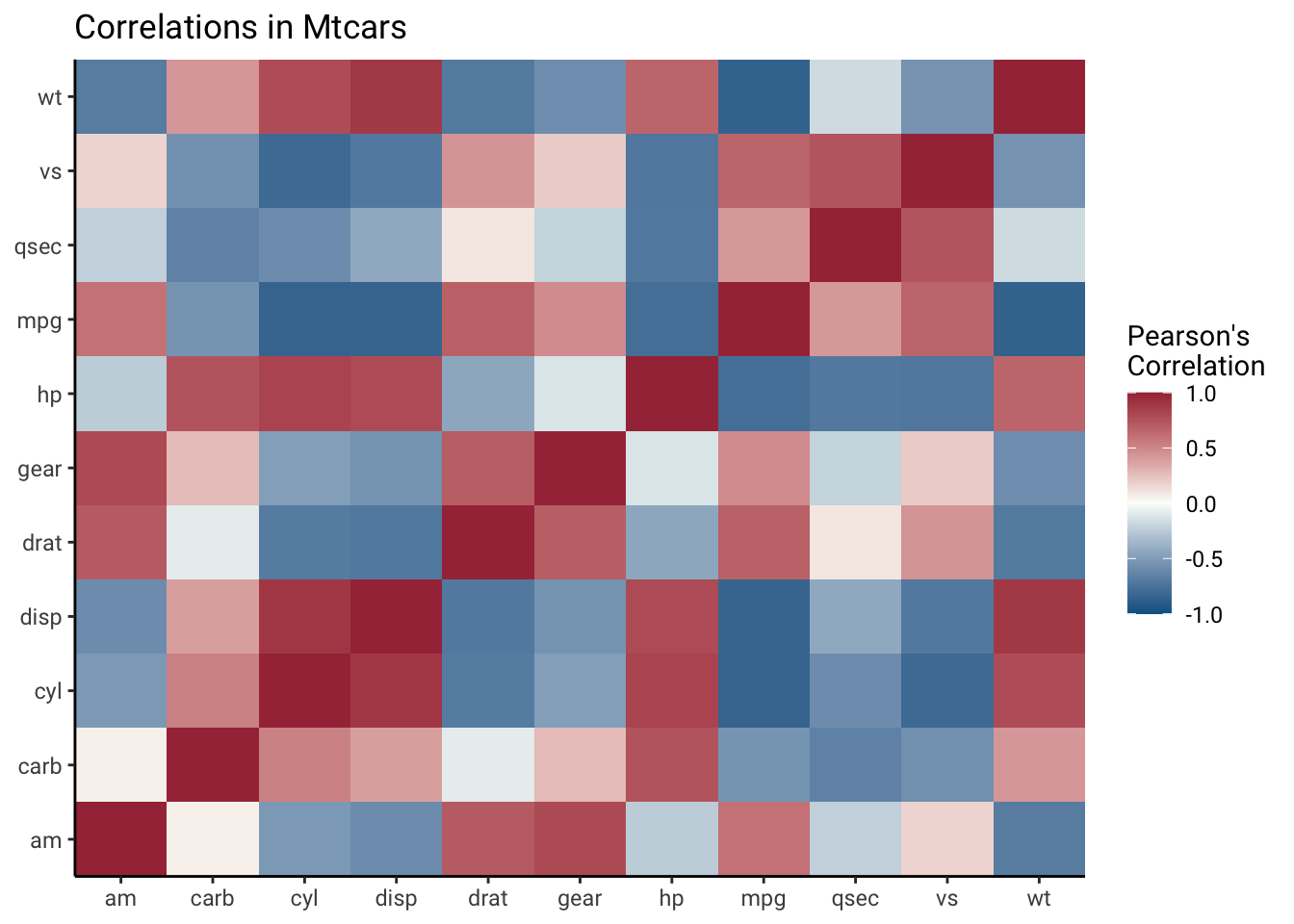We can add the correlations for extra information. For this particular plot, I only added significant (p-value less than 0.05) correlations, using the column `r_if_sig` that outputs from `formatted_cors()`.

``````formatted_cors(mtcars) %>%
ggplot(aes(measure1, measure2, fill=r, label=round(r_if_sig,2))) +
geom_tile() +
labs(x = NULL, y = NULL, fill = "Pearson's\nCorrelation", title="Correlations in Mtcars",
subtitle="Only significant Pearson's correlation coefficients shown") +
geom_text() +
theme_classic() +
scale_x_discrete(expand=c(0,0)) +
scale_y_discrete(expand=c(0,0)) +
theme(text=element_text(family="Roboto"))``````Another version of this could involve squares with different sizes to denote strength of correlation using `geom_point` with `shape` set to a value from these available `geom_shape`s. Make sure you take the absolute value of the correlation so that strong negative correlations can also be denoted larger.

``````formatted_cors(mtcars) %>%
ggplot(aes(measure1, measure2, col=r)) + ## to get the rect filled
geom_tile(col="black", fill="white") +
geom_point(aes(size = abs(r)), shape=15) +
labs(x = NULL, y = NULL, col = "Pearson's\nCorrelation", title="Correlations in Mtcars") +
theme_classic()+
scale_x_discrete(expand=c(0,0)) +
scale_y_discrete(expand=c(0,0)) +
theme(text=element_text(family="Roboto")) +
scale_size(range=c(1,11), guide=NULL) ``````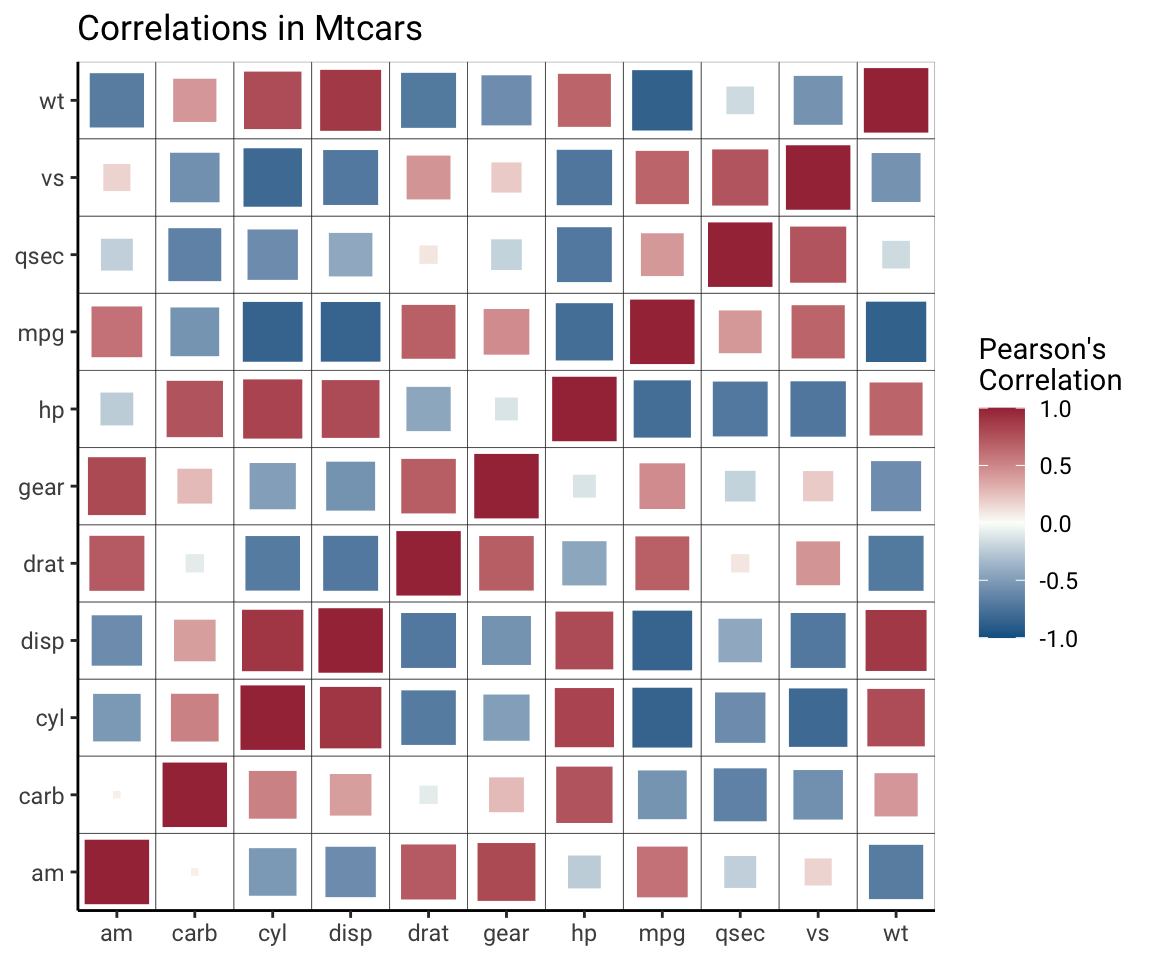Please feel free to reach out with questions or suggestions. Thank you to Elena Leib for spotting a minor bug in a previous version of this post!

# Just the code

``````cors <- function(df) {
M <- Hmisc::rcorr(as.matrix(df))
Mdf <- map(M, ~data.frame(.x))
return(Mdf)
}

formatted_cors <- function(df){
cors(df) %>%
map(~rownames_to_column(.x, var="measure1")) %>%
map(~pivot_longer(.x, -measure1, "measure2")) %>%
bind_rows(.id = "id") %>%
pivot_wider(names_from = id, values_from = value) %>%
rename(p = P) %>%
mutate(sig_p = ifelse(p < .05, T, F),
p_if_sig = ifelse(sig_p, p, NA),
r_if_sig = ifelse(sig_p, r, NA))
}

formatted_cors(mtcars) %>%
ggplot(aes(measure1, measure2, fill=r, label=round(r_if_sig,2))) +
geom_tile() +
labs(x = NULL, y = NULL, fill = "Pearson's\nCorrelation", title="Correlations in Mtcars",
subtitle="Only significant Pearson's correlation coefficients shown") +
geom_text() +
theme_classic() +
scale_x_discrete(expand=c(0,0)) +
scale_y_discrete(expand=c(0,0)) +
theme(text=element_text(family="Roboto"))``````### Session info

``sessionInfo()``

R version 4.1.3 (2022-03-10) Platform: x86_64-apple-darwin17.0 (64-bit) Running under: macOS Catalina 10.15.7

Matrix products: default BLAS: /Library/Frameworks/R.framework/Versions/4.1/Resources/lib/libRblas.0.dylib LAPACK: /Library/Frameworks/R.framework/Versions/4.1/Resources/lib/libRlapack.dylib

locale:  en_US.UTF-8/en_US.UTF-8/en_US.UTF-8/C/en_US.UTF-8/en_US.UTF-8

attached base packages:  stats graphics grDevices utils datasets methods base

other attached packages:  gt_0.6.0 knitr_1.38 forcats_0.5.1 stringr_1.4.1
 tibble_3.1.8 ggplot2_3.3.6 tidyverse_1.3.1

loaded via a namespace (and not attached):  fs_1.5.2 lubridate_1.8.0 RColorBrewer_1.1-3  httr_1.4.2 tools_4.1.3 backports_1.4.1
 utf8_1.2.2 R6_2.5.1 rpart_4.1.16
 Hmisc_4.7-0 DBI_1.1.2 colorspace_2.0-3
 nnet_7.3-17 withr_2.5.0 tidyselect_1.1.2
 gridExtra_2.3 cabinets_0.6.0 compiler_4.1.3
 cli_3.3.0 rvest_1.0.2 htmlTable_2.4.0
 xml2_1.3.3 labeling_0.4.2 sass_0.4.1
 scales_1.2.1 checkmate_2.0.0 digest_0.6.29
 foreign_0.8-82 rmarkdown_2.13 base64enc_0.1-3
 jpeg_0.1-9 pkgconfig_2.0.3 htmltools_0.5.2
 dbplyr_2.1.1 fastmap_1.1.0 htmlwidgets_1.5.4
 generics_0.1.3 farver_2.1.1 jsonlite_1.8.0
 magrittr_2.0.3 Formula_1.2-4 Matrix_1.4-0
 Rcpp_1.0.9 munsell_0.5.0 fansi_1.0.3
 lifecycle_1.0.1 stringi_1.7.8 yaml_2.3.5
 plyr_1.8.7 grid_4.1.3 crayon_1.5.1
 lattice_0.20-45 haven_2.5.0 splines_4.1.3
 hms_1.1.1 pillar_1.8.1 reshape2_1.4.4
 reprex_2.0.1 glue_1.6.2 evaluate_0.15
 latticeExtra_0.6-29 data.table_1.14.2 modelr_0.1.8
 png_0.1-7 vctrs_0.4.1 tzdb_0.3.0
 cellranger_1.1.0 gtable_0.3.0 assertthat_0.2.1
 xfun_0.32 broom_0.8.0 ggcorrplot_0.1.3
 survival_3.2-13 corrgram_1.14 cluster_2.1.2
 corrplot_0.92 ellipsis_0.3.2